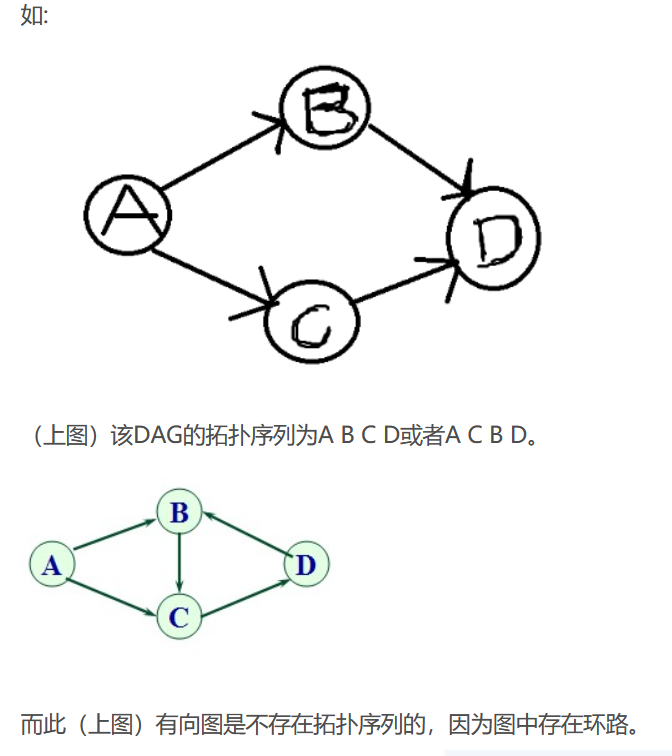# CF1027D Mouse Hunt（topo总结）

AC代码

#include<cstdio>
#include<iostream>
#include<algorithm>
using namespace std;
const int N = 2e5 + 20;
int n, m, cost[N], ans = 0, k, nex[N], du[N];
bool vis[N];

void Topo(int x)
{
vis[x] = true;
du[nex[x]]--;
if (!du[nex[x]]) Topo(nex[x]);
}

int Dfs(int x)
{
vis[x] = true;
if (!vis[nex[x]]) return min(Dfs(nex[x]), cost[x]);
else return cost[x];
}

int main()
{
cin >> n;
for (int i = 1; i <= n; i++)
cin >> cost[i];
for (int i = 1; i <= n; i++)
{
int x;
cin >> x;
nex[i] = x, du[x]++;
}
for (int i = 1; i <= n; i++)
if (!du[i] && !vis[i])
Topo(i);
for (int i = 1; i <= n; i++)
if (!vis[i]) ans += Dfs(i);
cout << ans << endl;
return 0;
}


1、拓扑排序在有向无环图中才能排出有效的序列，否则能判断该有向图有环。
2、如果输入的有向图中的点,不存在入度为0的点,则该有向图存在回路
3、如果存在的入度为0的点大于一个,则该有向图肯定不存在一个可以确定的拓扑序列但并不妨碍拓扑排序

1)只有有向无环图才存在拓扑序列;
2)对于一个DAG,可能存在多个拓扑序列;(1)从有向图中选取一个没有前驱(即入度为0)的顶点，并输出之;
(2)从有向图中删去此顶点以及所有以它为尾的弧;

#include<iostream>
#include<stdlib.h>
#include<stdio.h>
#define MAX 100
#include<cstring>
using namespace std;
//n:关联的边数，m:节点数
void toposort(int mp[MAX][MAX],int indegree[MAX],int m)
{
int i,j,k;
printf("该DAG的拓扑序列为：");
for(i=1;i<=m;i++) //遍历m次
{
for(j=1;j<=m;j++) //找出入度为0的节点
{
if(indegree[j]==0)
{
indegree[j]--;

cout<<j<<"  ";
for(k=1;k<=m;k++) //删除与该节点关联的边
{
if(mp[j][k]==1)
{
indegree[k]--;
}
}
break;
}
}
}
}
int main()
{
int n,m; //n:关联的边数，m:节点数
while(scanf("%d %d",&n,&m)==2&&n!=0)
{
int i;
int x,y;
int mp[MAX][MAX]; //邻接矩阵
int indegree[MAX]; //入度
memset(mp,0,sizeof(mp));
memset(indegree,0,sizeof(indegree));
for(i=1;i<=n;i++)
{
scanf("%d %d",&x,&y);
if(!mp[x][y])
{
mp[x][y]=1;
indegree[y]++;
}
}
toposort(mp,indegree,m);
}
return 0;
}

06-22
09-2512-13
01-15
08-24263
05-1417
10-0123
07-031243
03-2099
03-08220